# ISDN Basic Access

## Some explanations of terms

The most common type of ISDN connection is the  basic rate interface  $\rm (BRI)$,  which is used in particular by residential customers and medium-sized companies.

As shown in the diagram, an ISDN connection consists of the following functional groups:

•   Terminal Equipment Type 1  $\rm (TE1$,  ISDN terminal equipment$)$,
•   Terminal Equipment Type 2  $\rm (TE2$,  analog terminal equipment$)$,
•   Terminal Adapter  $\rm (TA)$,
•   Network Termination 1  $\rm (NT1)$,
•   Network Termination 2  $\rm (NT2$,  optional$)$,
•   Line Termination  $\rm (LT)$,
•   Exchange Termination  $\rm (ET)$.

The reference points between the individual function groups are designated

$\rm R$,  $\rm S$,  $\rm T$,  $\rm U$  $\rm V$.

This also results in the usual ISDN designations of various buses:

• $\rm S_{\rm 0}$  bus:   Reference point  $\rm S$  between subscriber line area and network termination; the  "$\rm 0$"  stands for basic rate interface.
• $\rm U_{\rm K0}$  bus:   Reference point  $\rm U$  between network termination and local exchange; the  "$\rm K$"  stands for  "copper cable",  the  "$\rm 0$"  again for  "basic rate interface".

The following sections describe this subscriber line configuration and the interfaces of the ISDN basic rate interface in detail.

## Logical channels

The basic rate interface consists of at least three channels, namely

• two  "user channels"  resp.  "bearer channels"   ⇒   "B channels"  with  $\text{64 kbit/s}$  each,  and
• one  "signaling channel"  resp.  "data channel"   ⇒   "D channel"  with  $\text{16 kbit/s}$.

⇒   A bearer channel is used for the transmission of user information  $($voice,  texts,  images,  data,  etc.$)$.  Two B channels can be active at the same time,  operate independently of each other and have different destination devices.

⇒   The D channel is mainly responsible for controlling the B channels.  In particular,  it is responsible for establishing and terminating connections and for communication control.

⇒   In addition,  the D channel can be used for  data transmission.  Due to the low data rate (16 kbit/s),  however,  this is only of interest for applications with very low data volumes,  for example,  monitoring systems or online booking systems.

⇒   The graphic shows the logical channels of the ISDN basic rate interface.  With

• the two user channels  (64 kbit/s each),  and
• the combined data and signaling channel  (16 kbit/s)

the subscriber has a total net bandwidth of  $\text{144 kbit/s}$  at his disposal.

⇒   All logical channels are bidirectional.  The lower part of the graphic shows the implementation in the basic rate interface  $\rm (BRI)$:

• Between the exchange and the network termination – i.e. on the  $\rm U_{\rm K0}$ bus – there is a  two-wire connection  that is operated in both directions.
• In contrast,   four-wire transmission  is used in the house connection area – on the  $\rm S_{\rm 0}$ bus – whereby one twisted pair is required for each of the two directions.

## Terminal equipment for ISDN

There are two different types of terminal equipment for ISDN:

• Terminal Equipment Type 1  $\rm (TE1)$:   This includes,  for example,  ISDN-capable telephones,  ISDN group 4 fax devices and ISDN PC cards.  These terminal equipment are connected directly to the  $\rm S_{\rm 0}$  interface via the network termination  $\rm (NTBA)$.

• Terminal Equipment Type 2  $\rm (TE2)$:  This refers to terminal equipment for the analog telephone network  (telephones, group 3 fax machines and conventional modems)  that must be connected to the   $\rm S_{\rm 0}$ interface via a terminal adapter.

• Terminal Adapter  $\rm (TA)$:  This allows TE2 devices to be used with ISDN.  Such adapters can have analog interfaces to the terminal  (so-called a/b interfaces)  or digital interfaces,  for example for connecting terminals via V.24 or X21.

$\text{Example 1:}$  The graphic shows a possible ISDN configuration.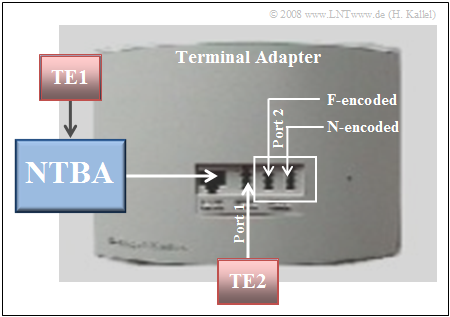Configuration for an ISDN basic rate interface

In the center is the terminal adapter  "TA2a/b Komfort"  from Deutsche Telekom,  which is part of the uni@home package.  This has two integrated  a/b ports  with three TAE sockets for analog terminals:

• Port 1 is universal coded;  one really speaks of  "encoding"  here,  even if this pin assignment has nothing to do with an encoding in communications.  TE2 terminals of any kind  $($telephone, fax, modem, etc.$)$  can be connected to this port.

• Port 2 consists of an N-encoded TAE socket such as fax,  answering machine,  etc.  and an F-encoded socket for a telephone.  (The  "N"  here stands for  "non-voice devices").  For port 2,  only one of the two inputs can be active at the same time.

## General description of the S0 interface

The  $\rm S_{\rm 0}$  bus is an in-house bus and represents the connection between the network termination adapter  $\rm (NTBA$  or  $\rm NT)$  and the ISDN terminal equipment  $\rm (TE)$.

Up to eight terminal equipment such as telephones,  fax machines,  ISDN cards and also ISDN private branch exchanges can be connected simultaneously to a maximum of twelve connection sockets.

Such an  ISDN multiple subscriber line  has:

• three Multiple Subscriber Numbers  $\rm (MSN)$ – expandable up to ten,
• two user channels  ("B channels")  with 64 kbit/s each for the various services,
• one signaling or data channel with 16 kbit/s,
• twelve connection sockets with up to eight connected devices  (but only four voice terminals).

From the graphic you can see:

1. Of a four-core copper cable,  two cores  (one twisted pair)  are used to transport data from the terminal equipment  $\rm (TE)$  to the  $\rm NTBA$  $($"transmission line"$)$  and the other twisted pair is used to transfer data from the NTBA to the TE  $($"reception line"$)$.
2. Please note that in some technical articles the direction  "TE → NTBA"  is referred to as the reception line and the opposite direction  "NTBA → TE"  as the transmission line.  This different description compared to our tutorial results from the view of the NTBA.
3. The  $\rm S_{\rm 0}$  bus provides two bearer channels  $($B1 and B2$)$  for user data transmission with 64 kbit/s each and additionally the data channel with 16 kbit/s for signaling during connection setup and for various synchronization data.
4. This results in a   net transmission rate of  $\text{144 kbit/s}$   per direction for the  $\rm S_{\rm 0}$ bus.  The  gross transmission rate,  taking control bits into account,  is  $\text{192 kbit/s}$.

Several terminals can be connected in parallel to the  "Network Termination for ISDN Basic Rate Access"  $\rm (NTBA)$  via the   $\rm S_{\rm 0}$ bus.

• The open line ends must be terminated with the wave impedance of  $\rm 100\ Ω$  $($real$)$,  otherwise reflections will occur.
• The  $\rm S_{\rm 0}$ bus generally reacts tolerantly to mismatching,  but in this case certain length limitations and installation rules must be observed.
• The possible cabling types for the  $\rm S_{\rm 0}$ bus in the multi-device connection operating mode are shown in the graphic on the right.

⇒   The usual connection type is the  short bus  with a maximum length of  $150$  meters.  Up to eight terminal equipment – distributed as required – can be connected to this bus.

⇒   The  extended bus  is at least  $100$  meters and at most  $500$  meters long.  A maximum of four terminal equipment can be connected,  but these may only be positioned within the last  $50$  meters before the terminating impedance.

⇒   For a point-to-point connection,  the cable length can be up to  $1000$  meters.  In this case,  it is referred to as a  long bus.  It should be noted,  however,  that only one terminal equipment can be connected at this length  $($"single connection"$)$.

## Telecommunications aspects of the S0 interface

On the  $\rm S_0$ bus,  the two bearer channels and the data channel are transmitted together with synchronization and control bits in frames of  $48$  bits each in time division multiplex.  The  modified AMI code  $($the name stands for "alternate mark inversion"$)$  is used,  which can be characterized as follows:

1. The logical  (binary)  "1"  is represented by the voltage value  $0\ \rm V$  and the logical  (binary)  "0"  alternately by   $+s_0$   or   $-s_0$,  as the comparison of the upper and lower signal waveforms in the graphic of  $\text{Example 2}$  shows.  For ISDN,  the modulation range  $s_0 = 0.75\ \rm V$  is fixed.
2. The pseudo-ternary coding ensures that the  $\rm S_0$  bus remains free of DC voltage at all times.  The redundancy of   $1 - 1/\log_2 (3) ≈ 37\%$   is used in ISDN,  for example,  for frame synchronization by deliberately violating the AMI coding rules to mark frames.
3. The  $\rm S_0$ bus is writable by the logical  "AND"  operation.  This means that the NTBA only receives the logical  "1"  if all terminal devices equipment send a logical  "1" - i.e. the zero level.  If only one device sends the logical  "0",  this is also received.
4. All terminal equipment obtains its clock from the NTBA and operates bitsynchronously. The quiescent signal is always the zero level,  i.e. the logical  "continuous one".  In addition,  since all devices in the data channel always transmit a  "0"  with same polarity,  pulses cannot cancel each other out.

$\text{Example 2:}$  The graphic illustrates the difference between the  "modified"  and the  "conventional AMI code",  namely the swapping of  "0"  and  "1"  in the binary signal.  The reason for this interchange is:

• With the conventional AMI code,  no symbols are transmitted during a speech pause either.  Or in other words,  only pulses with the amplitude  $0 \ \rm V$  are transmitted over a longer period of time  $($see middle diagram$)$.  This makes clock synchronization more difficult.

• With the modified AMI code  $($lower diagram$)$,  positive and negative pulses alternate   ⇒   simple clock synchronization at  "duration zero".  Critical is now a  "continuous one"  signal,  but this occurs in voice transmission never  (or only extremely rarely).

We received this note on the practical significance of the modified AMI code from Prof. Peter Richert  (FH Münster).  Many thanks!

## Frame structure of the S0 interface

The frame structure of the  $\rm S_0$ interface - i.e. the bus between the network termination  $\rm (NTBA)$  and the subscriber terminal equipment  $\rm (TE)$  - is shown in the upper part of the following graphic.  The lower part shows the frame structure in the opposite direction  $\rm (TE → NTBA)$.

Each frame consists of

• $2 · 8 = 16$  bits for each of the two bearer channels  $(32$  bits in total$)$,
• $4$  bits for the data channel  (marked green),  and
• another  $12$  control bits,  which are different for the two transmission directions.

Each frame is thus composed of  $48$  bits,  which are transmitted in  $250$  microseconds  $(4000$  frames per second$)$.

This results in a  total gross bit rate  of  $48 · 4000 = 192\ \rm kbit/s$.

The  twelve bits for control information  are composed as follows:

• $\rm A$ bit:  Activation bit  (marked in brown),
• $\rm E$ bits:  Bits for echo channel  (marked red),
• $\rm F$ bit:  Frame synchronization bit,
• $\rm FA$ bit:  Additional frame synchronization bit,
• $\rm L$ bits:  DC equalization bits,
• $\rm N$ bit:  Inverted  $\rm FA$  bit,
• $\rm S$ bits:  Reserved for future applications.

The interaction of these control bits is described in  $\text{Example 3}$.

$\text{In advance only so much:}$

1. Each frame is composed of subframes,  whereby each subframe – and thus the entire frame - must be kept DC–free.
2. To achieve this,  so-called  $\rm L$ bits  are used in each subframe.
3. Such a  $\rm L$ bit  is always logical  "0"  with positive or negative polarity  $(±0.75 \ \rm V)$,  if the number of polarity changes after the last  $\rm L$ bit  is odd.
4. On the other hand,  if the number of polarity changes is even,  the   $\rm L$ bit  is  logical  "1"   ⇒   $0 \ \rm V$.

$\text{Example 3:}$  The following description of the bit assignment  $($depending on the transmission direction$)$  is based on the above graphic for the  $\rm S_0$ frame structure.

⇒   In  both directions

1.   The first bit of each frame is the frame synchronization bit  $(\rm F$  bit$)$.  This is always  "0"  with positive polarity   ⇒   $+0.75 \ \rm V$.
2.   This is followed by a DC compensation bit  $\rm (L$  bit:  "0"  with negative polarity   ⇒   $-0.75 \ \rm V)$  to prevent a DC component.
3.   Since an  $\rm L$  bit  $($with level  $+0.75 \ \rm V)$  has also occurred at the end of the last frame,  the  $\rm F$  bit of the current frame leads to a deliberate violation of the AMI coding rule  $($purple marking in the graphic$)$,  which is used for frame synchronization.
4.   The 14th bit is an additional frame synchronization bit  $(\rm FA$  bit$)$  which is always set to  "0 negative"  $(-0.75 \ \rm V)$.
5.   Since the subframe between bits 3 and 13 is always DC-free, the  $\rm FA$  bit  also generates an intentional code violation together with the  $\rm L$.
6.   This second code violation confirms the code violation originating from the  $\rm F$  bit.  This prevents a transmission error from being incorrectly interpreted as a frame start.
7.   Loss of frame synchronization is assumed when no code violation pairs are identified within two frames.
8.   Frame synchronization is complete when three consecutive code violations have been detected:   "$\rm F – FA – F$".

⇒   Different terminal equipment can transmit simultaneously in the  transmitting direction  (lower graphic):

1.   Each device must be DC-free by the respective  $\rm L$  bit after each bearer channel  $($bit 11, 24, 35, 46$)$  and data channel  $($bit 13, 26, 37, 48$)$.
2.   The $\rm L$  bit at position 15 is always  "0"  with positive polarity  $(+0.75 \ \rm V)$.

⇒   In the  reception direction  (upper graphic),  only the NTBA transmits and thus a single compensation bit  $\rm L$  bit$)$  is sufficient except for bit 2 at the end.  In addition,  there are further control bits in this direction  $\rm (NTBA → TE)$:

1.   The thirteenth bit is the activation bit  $(\rm A$  bit$)$.  This is set to  "0"  when activated and to  "1"  when deactivated.
2.   The  $\rm N$  bit at bit position  15  always directly follows the  $\rm FA$  bit  and is inverted with respect to it,  i.e. set to  "1"   ⇒   $0 \ \rm V$.
3.   The bits  $\rm S1$  and  $\rm S2$  are reserved for future applications and are currently always set to logical  "0"  $($with different polarity$)$.
4.   The total of four  $\rm E$  bits  (for the echo channel)  in the reception direction depend on the data bits of the transmission direction.
5.   These bits can be used to decide which terminals are currently allowed to transmit.  This will be discussed in detail in the next section.

Finally,  it will be shown how ISDN allows several terminals to  access the data channel  without collisions,  and which terminal has priority when transmitting.

The  $\rm C$arrier $\rm S$ense $\rm M$ultiple $\rm A$ccess  $\rm (CSMA)$  method is used for blocking and unblocking the data channel and can be described briefly as follows:

• The  echo channel  is inserted into the data stream for the direction  $\rm NTBA → TE$.  This is the totality of the  $\rm E$  bits marked in red in the graphic  $($on the previous section$)$,  each of which transmits a previously received  $\rm D$  bit back to the terminals.
• An    $\rm E$  bit  results from the  AND operation  of all participants.  This means:  In the echo channel,  the logical  "1"  only occurs if every participant reports a  "1"  - i.e. sends nothing.  A  "0"  in the echo channel indicates that a device has access to the data channel or at least wishes to do so.
• Each terminal equipment checks whether the data channel is free or busy by  listening on the echo channel.  For this purpose,  the terminal waits for at least  $N$  consecutive logical ones  $(0 \ \rm V)$  in the echo channel.  For a telephone device  $N = 8$,  for data devices  $N = 10$.
• After  $N$  ones,  one can assume with sufficient certainty that there is a free data channel and the device starts to transmit.  For transmission security,  each piece of user information starts and ends with the bit pattern  "01111110"   ⇒   $\rm (flag)$  according to the protocol of layer  2  of the OSI reference model.

An example shows which terminal equipment is given priority when two terminal equipment want to start transmitting at the same time.

$\text{Example 4:}$  Two terminal devices  $\rm TE1$  and  $\rm TE2$  want to access the data channel at the same time.  They both wait for  $N = 8$  consecutive logical ones on the echo channel $($marked blue in the graphic, time marker  $t_1)$.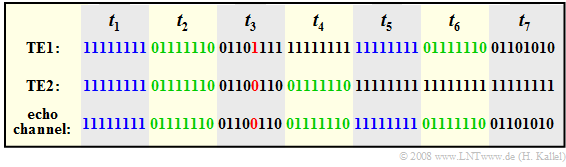Data channel access and interaction with the echo channel
1.   After that,  both send  – again simultaneously –  a flag  $($green mark,  time marker  $t_2)$.
2.   At time  $t_3$,  both terminals send their information.  In the echo channel,  a collision is detected if the bits of TE1 and TE2 differ.
3.   The logical  "0"  has priority over the logical  "1".  In the example,  TE2 has priority over TE1,  because the fifth bit of TE2 is a  "0"  and the fifth bit of TE1 is a  "1"  $($red marks$)$.
4.   Thus TE1 will stop the transmission.  It can only send its information at time  $t_7$  after TE2 completes its transmission with a flag  $(t_4)$,  and thus the data channel is free again from  $t_5$.
5.   TE1 checks this at time marker  $t_5$  and sends a flag again afterwards  $(t_6)$.

## General description of the UK0 interface

According to the  "ISO-OSI reference model",  the  $\rm U$ interface is generally the connection between the network termination  ("Network Termination for ISDN Basic rate Access",  $\rm NTBA)$  and the line termination  $\rm (LT)$  in the exchange.

• In the case of the ISDN basic rate interface,  this point is called the  $\rm U_{K0}$ interface and the associated bus the  $\rm U_{K0}$ bus.  "$\rm K$"  stands here for the transmission medium  "copper"  and  "$0$"  for the basic rate interface.
• The line termination  $\rm (LT)$  forms the transmission-technical termination on the network side.  It handles all switching and operational tasks,  line coding,  transmission control,  remote power supply,  and test and monitoring functions.

The graphic shows the  $\rm U_{K0}$ interface as the network access for a subscriber.  This interface  $($or the associated bus$)$  is characterized by the following features: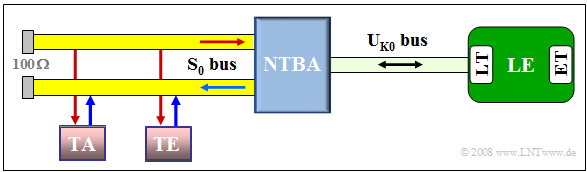ISDN basic rate interface with NTBA,  $\rm S_{0}$ bus  and  $\rm U_{K0}$ bus

1.   The  $\rm U_{K0}$ bus is implemented by two-wire transmission over a single copper twin wire,  with ranges of  $8$  kilometers  $($with  $0.6$  millimeter diameter)  or  $6$  km  (with  $0.4$  mm diameter)  possible.
2.   The gross data transmission rate is  $\text{160 kbit/s}$  in both directions, distributed over two user channels of  $\text{64 kbit/s}$  each,  the data channel with  $\text{16 kbit/s}$  and an additional  $\text{16 kbit/s}$  channel for synchronization and service.
3.   In contrast to the  $\rm S_0$ bus  $($modified AMI code$)$,  a line code with less redundancy is used here.  In Germany and Belgium,  a  $\rm 4B3T$ code  is used  (see next section),  in other European countries the redundancy-free quaternary code  $\rm (2B1Q)$.
4.   In the 2B1Q code,  two binary symbols are mapped to one quaternary symbol each;  the four possible voltage values are  $±2.5 \ \rm V$  and  $±0.83 \ \rm V$.
5.   The advantage is the smaller symbol rate of  $80000$  quaternary symbols per second  $\text{(80 kBaud)}$   ⇒   a larger operating range.
6.   The disadvantage is that transmission errors cannot be detected due to the lack of redundancy and that the 2B1Q code is not DC-signal-free.

## Telecommunications aspects of the UK0 interface

In the ISDN network of  "Deutsche Telekom",  the   $\rm MMS43$  code   ⇒   "$\rm M$odified $\rm M$onitored $\rm S$um $4$B$3$T"  is used for transmission on the  $\rm U_{K0}$ bus.

The graphic below shows the four code tables of the MMS43 code.  In the following, only the most important properties will be summarized:

1. In each 4B3T code, four binary symbols  $\rm (4B)$  are represented by three ternary symbols  $\rm (3T)$,  which are simplified here as "–",  "0"  and  "+".  These symbols represent the signal levels  $–2.5 \ \rm V$,  $0 \ \rm V$  and  $+2.5\ \rm V$.  The ternary decision has two thresholds.
2. Compared to the binary code and also compared to the AMI code,  the symbol rate is  $25\%$  smaller.  This means:   The bit rate  $160 \ \rm kbit/s$  leads here to the symbol rate  $120 \ \rm kBaud$  $(120 \hspace{0.08cm}000$  ternary symbols per second$)$  and not to  $160 \ \rm kBaud$  as on the  $\rm S_0$ bus.
3. The symbol rate is larger than for the 2B1Q code  $(80 \ \rm kBaud)$.  However,  a significant advantage of the redundant 4B3T code is that it is free of DC signals.  This means that a constant voltage value of  $+2.5\ \rm V$  or  $-2.5\ \rm V$  cannot occur over a longer period of time.
4. Another characteristic of the MMS43 code is that four different code tables are available for the conversion from binary to ternary,  which are selected according to the running digital sum  ${\it Σ}_l$  $($of the ternary values$)$.  The graph shows these tables.
5. Six of the total  $16$  entries  $($marked by red arrows$)$  are the same in all four lines and thus apply independently of the running digital sum  $\rm (RDS)$.  These ternary sequences each contain a  "+"  and a  "–",  so that the RDS value is preserved:   ${\it Σ}_{l+1} = {\it Σ}_l$.
6. Let us now consider a binary word with different entries:   If   ${\it Σ}_l= 0$,  the binary sequence "0000" is replaced by  "+ 0 +",  increasing  $\rm RDS$  by  $2$.  In all the other cases, "0 – 0" is output,  decreasing  $\rm RDS$  by  $1$.
7. The two lines of the table for  ${\it Σ}_l= 1$  and  ${\it Σ}_l= 2$  differ only in two entries,  which are highlighted in the graphic by blue frames.  This is the only difference between the MMS43 code and the conventional MS43 code,  which makes do with only three code tables.

## Frame structure of the UK0 interface

Each frame on the  $\rm U_{K0}$ bus is made up of  $120$  ternary symbols and is transmitted in one millisecond. This results in a  symbol rate of  $120 \ \rm kBaud$.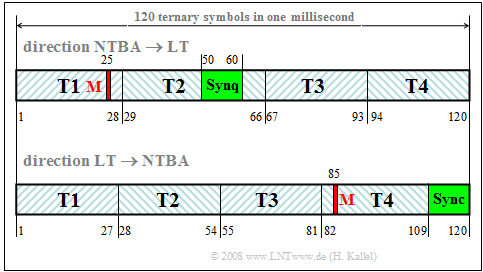Frame structure of the  $\rm U_{K0}$ interface

The graphic illustrates the  $\rm U_{K0}$ frame structure,

1. in the upper part in the direction from the network termination  $\rm (NTBA)$  to the  line termination  $\rm (LT)$  in the local exchange,
2. below in the opposite direction,  from  $\rm (LT)$  to   $\rm (NTBA)$.

It can be seen:

• The information symbols are embedded in four subframes  $\rm T1$, ... ,  $\rm T4$  of  $27$  ternary symbols each,  which result from  $36$  input bits according to the 4B3T coding.
• These  $36$  bits are composed of  $16$  bits each of the two bearer channels  $\rm B1$  and  $\rm B2$  and four bits of the data channel,  which are interleaved.
• Furthermore,  there is a synchronization word  $(\rm Sync)$  derived from the Barker code with eleven ternary symbols that differ in position and content for the two directions.
• The  "maintenance symbol"   $\rm M$  (red marking)  is derived from eight overframes and is mainly used for service purposes.

## Network termination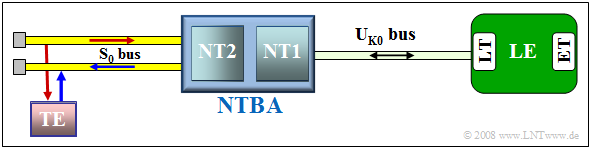Network termination device  $\rm (NTBA)$  as a connection between  $\rm S_0$ and   $\rm U_{K0}$ bus

The  $\rm NTBA$  $(N\hspace{-0.05cm}$etwork  $T\hspace{-0.05cm}$ermination  for  ISDN  $B\hspace{-0.05cm}$asic  Rate  $A\hspace{-0.05cm}$ccess$)$

• connects an in-house ISDN terminal equipment  $\rm (TE)$  to the higher-level local network via the exchange,
• implements the conversion from the ISDN access network,  which has two wires at the NTBA,  to the four-wire house access line.
• is an extremely important ISDN network component,  as it enables the transition between the  $\rm S_0$ bus and the  $\rm U_{K0}$ bus.

In general,  the NTBA is composed of two parts called  "NT1"  and  "NT2".

The tasks of the mandatory termination  $\rm NT1$  are:

1. Conversion from two-wire to four-wire  (and vice versa)  and the code conversion between 4B3T code  $(\rm U_{K0})$  and AMI code  $(\rm S_0)$,
2. Power supply for the  $\rm S_0$ bus  $(230\ \rm V$, max. $4.5\ \rm W$  power output$)$,  whereby the NT1 is supplied by the exchange via the  $\rm U_{K0}$ interface during normal operation,
3. Power supply of up to four terminal equipment of the  $\rm S_0$ interface in emergency operation;  for this purpose,  the NT1 must be connected to a safe power supply,
4. Execution of all operating functions;  this includes,  among other things,  the detection of frame errors and the execution of tests and so-called  "loop formations".

The optional  $\rm NT2$  is only needed if a private branch exchange is to be connected behind the network termination:

1. In the  "Deutsche Telekom" network,  however, up to eight terminals  (maximum four ISDN telephones)  can be connected via the passive  $\rm S_0$ bus.
2. In this case,  the NT2 can also be dispensed with in the extension configuration.  The NTBA then consists only of the NT1.

## Directional separation method

Because of its transmission characteristics  $($long transmission range,  low bandwidth$)$, the  full-duplex frequency-division method  is used on the  $\rm U_{K0}$ bus,  i.e.  the signals are transmitted in both directions via a two-wire line at the same time and in the same frequency band.

The signals of the transmitting and receiving directions are separated from each other by means of a  fork circuit,  as shown in the diagram.

⇒   The realization is explained in detail in  $\text{Example 5}$.  Here only a short explanation of the operations using the example of subscriber  $\rm A$:

• At receiver  $\rm A$,  only the signal  $r_{\text{A}}(t)$  should arrive,  which would without noise,  distortions and echoes be equal to the transmitted signal  $s_{\text{B}}(t)$  from subscriber  $\rm B$  $($component shown in red$)$.
• However,  the signal  $r_{\text{A}}(t)$  is now superimposed by the signal  $s_{\text{A}}\hspace{-0.03cm}'(t)$,  which reaches the receiver via the fork circuit from its own transmitter.  This is indicated by the blue arrow.
• The task of the fork circuit is now to keep this component  $s_{\text{A}}\hspace{-0.03cm}'(t)$  of the received signal as low as possible.

⇒  This is generally very successful for narrowband transmitted signals – for example speech – but not for a broadband signal.  In this case,  the   echo cancellation method   must also be used  $($see green blocks in the above graphic$)$.

Its mode of operation can be described very briefly as follows:

• The transmitter regularly emits  "test signals"  and measures the respective incoming signal,  which on the one hand originates from subscriber  $\rm B$  via the fork circuit,  but on the other hand also reaches its own receiver via near-end crosstalk.
• From the determined  "echo impulse response",  the echo canceller calculates the expected echo in normal operation of its own transmitter and subtracts this from the received signal.  The echo canceller can be implemented,   for example, by a "transversal filter"  whose coefficients are set and re-adjusted by a processor.

$\text{Example 5:}$  The graphic shows a common realization of the  fork circuit. One can see

• on the left,  the transmitted and received line of the  $\rm S_0$ interface,  and
• on the right,  the two-wire connection of the bidirectional  $\rm U_{K0}$ interface.

The task of the fork circuit is

• to switch the  (blue)  transmitted signal  $s_{\text{A} }(t)$  from the  $\rm S_0$ to the  $\rm U_{K0}$ side,
• and the  (red)  received signal  $r_{\text{A} }(t)$  in the opposite direction.

Care must be taken that the  $\rm S_0$ receiver is as completely decoupled as possible from the  $\rm S_0$ transmitter.  That means:  The signal component  $s_{\text{A} }\hspace{-0.03cm}'(t)$  with a gray background on the received line should disappear or at least be very small.

This circuit works in the following way:

1. $Z_{\rm L}(f)$  is the input impedance of the copper line of the ISDN access network coupled via the transformer.
2. $Z_{\rm N}(f)$  is the artificial line replica in the hybrid circuit.
3. If  $Z_1/Z_{\rm N}(f) = Z_2/Z_{\rm L}(f)$,  then  $s_{\text{A} }\hspace{-0.03cm}'(t) = 0$.
4. Here  $Z_1$  and  $Z_2$  denote real impedances.
5. $Z_{\rm L}(f)$  and  $Z_{\rm N}(f)$  are complex and thus frequency-dependent.

⇒   For narrowband signals,  the replication of  $Z_{\rm L}(f)$  by  $Z_{\rm N}(f)$  succeeds relatively well,  so that  $s_{\text{A} }\hspace{-0.03cm}'(t)$  can already be made sufficiently small by the fork circuit.  In contrast,  this is not possible for the entire frequency range in the case of broadband signals.  Here,  the echo cancellation must be applied additionally.

## Hierarchy of exchanges

An  exchange  provided the switching technology for the telephone network and for the logical and physical connection process between the subscriber lines.  One distinguished between several hierarchical levels.  The graphic summarizes this hierarchical structure.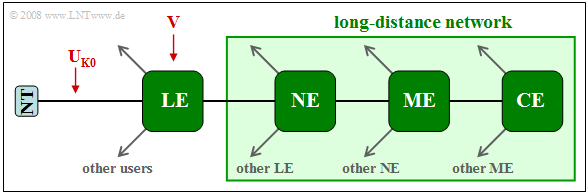Hierarchical structure of exchanges  $($historical$)$;  certainly not completely valid in 2018
• The  local exchanges  $\rm (LE)$  formed the lowest level.  An LE realizes the reference point  $\rm V$  of the network,  managed up to  $100,000$  ISDN subscribers,  fulfilled all switching functions and is the entry into the  "long-distance network".
• Above this were  node exchanges  $\rm (NE)$,  which were meshed together and connected to various main exchanges.  In Deutsche Telekom's territory,  there were approximately  $620$  node exchanges.
• The next level was formed by the  main exchanges  $\rm (ME)$,  of which there were about  $50$  in the Federal Republic of Germany.  These were also connected to each other and to the hierarchy highest level.
• The  central exchanges  $\rm (CE)$  formed the top hierarchical level of the long-distance network and served large regional areas. Each CE was also connected to an international exchange.